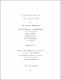## Minimal supersymmetric b-l and left-right models2016
##### Publisher
University of Alabama Libraries
##### Abstract

We propose a simple gauged U(1)B−L extension of the minimal supersymmetric Standard Model (MSSM), where R-parity is conserved as usual in the MSSM. The global B−L (baryon minus lepton number) symmetry in the MSSM is gauged and three MSSM gauge-singlet chiral multiplets with a unit B −L charge are introduced, ensuring the model free from gauge and gravitational anomalies. We assign an odd R-parity for two of the new chiral multiplets The scalar component of the R-parity even superfield plays the role of a Higgs field to beak the U (1)BL symmetry through its negative mass squared which is radiatively generated by the renormalization group running of soft supersymmetry (SUSY) breaking parameters. Because of our novel R-parity assignment, three light neutrinos are Dirac particles with one massless state. Since R-parity is conserved, the lightest superpartner (LSP) neutralino is a prime candidate of the cosmological dark matter. In particular, the B − L gauge boson (Z ), once discovered at the Large Hadron Collider, will be a novel probe of the Dirac nature of the light neutrinos since its invisible decay processes include the final states with one massless (left-handed) neutrino and two Dirac neutrinos, in sharp contrast with the conventional B − L extension of the SM or MSSM, where the right-handed neutrinos are heavy Majorana particles and decay to the SM leptons. We generalize a variation to the SUSY Left-Right symmetric model based on the gauge group SU (3)c × SU (2)L × SU (2)R × U (1)BL . The charge conjugate SU (2)L singlets are put into SU (2)R doublets, mirroring the former. We only introduce a second Higgs bidoublet to produce realistic fermion mass matrices. We calculate renormalization group evolutions of soft SUSY parameters at the one-loop level down to low energy. We find that an SU (2)R slepton doublet acquires a negative mass squared at low energies, so that the breaking of SU (2)R × U (1)BL → U (1)Y is realized by a non-zero vacuum expectation value of a right-handed sneutrino. Small neutrino masses are produced through neutrino mixings with gauginos. Mass limits on the SU (2)R × U (1)BL sector are obtained by direct search results at the LHC as well as lepton-gaugino mixing bounds from the LEP precision data.

##### Description
Electronic Thesis or Dissertation
Particle physics